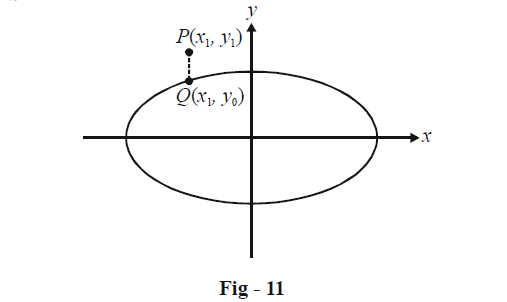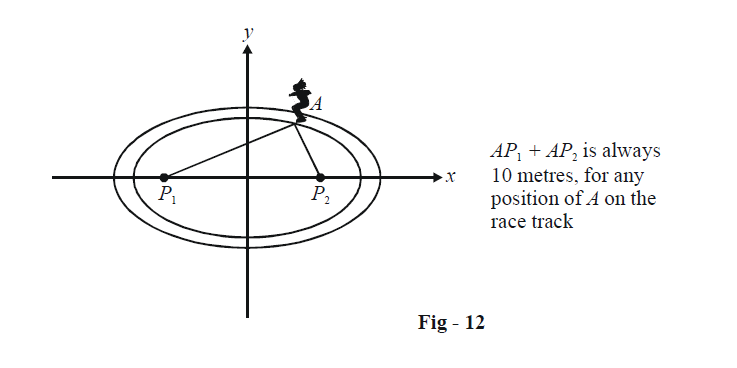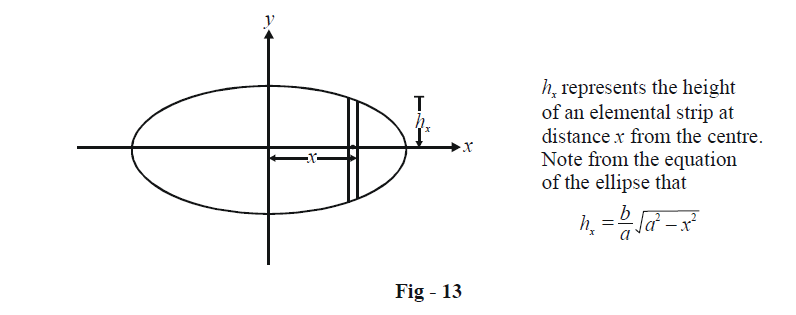# Basic Examples On Ellipses Set-2

Go back to  'Ellipse'

Example - 4

Given an ellipse  \begin{align}\S(x,\,\,y):\frac{{{x^2}}}{{{a^2}}} + \frac{{{y^2}}}{{{b^2}}} - 1 = 0\end{align} and a fixed point $$P({x_1},\,\,{y_1}),$$ how will we determine whether P lies inside or outside the ellipse ?

Solution: The approach we will follow here is the same as the one we used in circles and parabolas to solve the same question. Suppose P lies external to the ellipse as shown below. Drop a vertical line from P intersecting the ellipse at Q.Since Q lies on the ellipse, we have

\begin{align}&\frac{{x_1^2}}{{{a^2}}} + \frac{{y_0^2}}{{{b^2}}} = 1\\&\Rightarrow y_0^2 = {b^2}\left( {1 - \frac{{x_1^2}}{{{a^2}}}} \right)\end{align}

Since P lies external to the ellipse, we must have $$\left| {{y_1}} \right| > \left| {{y_0}} \right|$$ so that

\begin{align}&\qquad\; y_1^2 > y_0^2\\&\Rightarrow \quad y_1^2 > {b^2}\left( {1 - \frac{{x_1^2}}{{{a^2}}}} \right)\\&\Rightarrow \quad \frac{{x_1^2}}{{{a^2}}} + \frac{{y_1^2}}{{{b^2}}} - 1 > 0\\&\Rightarrow \quad S({x_1},\,\,{y_1}) > 0\end{align}

This condition must be satisfied if P lies outside the ellipse. (Convince yourself that wherever P may be outside the ellipse, this condition must hold). Similarly, P lies inside the ellipse if $$S({x_1},\,\,{y_1}) < 0.$$ We can write all this concisely as

$\boxed{\rm S({x_1},\,{y_1}) < 0 \Rightarrow P({x_1},\,{y_1}){\text{ lies inside the ellipse}} \\S({x_1},\,{y_1}) = 0 \Rightarrow P({x_1},{y_1}){\text{ lies on the ellipse}} \\S({x_1},\,{y_1}) > 0 \Rightarrow P({x_1},{y_1}){\text{ lies outside the ellipse}} }\\$

Example - 5

An athlete running around a race track finds that the sum of his distances from two flag posts is always 10 meters while the distance between the flag posts is 8 meters. What is the area that the race track encloses?

Solution:From the situation described, the race track must be an ellipse. The eccentricity is simply

$e = \frac{8}{{10}} = \frac{4}{5}$

(Refer to Page -5 to understand how to find eccentricity in such a scenario).

If the major axis is of length 2a, we have

${P_1}{P_2} = 2ae = 8$

$\Rightarrow \qquad a = 5$

Thus, $$b = a\sqrt {(1 - {e^2})} = 3.$$ The equation of the elliptical race track is

$\frac{{{x^2}}}{{25}} + \frac{{{y^2}}}{9} = 1$

To evaluate the area enclosed, we solve the general problem:

What is the area enclosed by the ellipse \begin{align}\frac{{{x^2}}}{{{a^2}}} + \frac{{{y^2}}}{{{b^2}}} = 1\,\,\,?\end{align}

To find the area, we divide the ellipse into elemental strips of width dx: one such strip is shown below.The area of the elemental strip shown is

\begin{align}&dA= 2{h_x}dx\\&{\rm{ }}\; \quad= \frac{{2b}}{a}\sqrt {{a^2} - {x^2}} dx\end{align}

The area of the right half of the ellipse is therefore

\begin{align}&{A_{{\rm{half}}}} = \frac{{2b}}{a}\int\limits_0^a {\sqrt {{a^2} - {x^2}} dx} \\&{\rm{ }}\qquad = \frac{\pi }{2}ab\end{align}

The total area of the ellipse is thus

$A = 2 \times {A_{{\rm{half}}}} = \pi ab$

For the current example, the area becomes

$A = \pi \times 5 \times 3 = 15\pi \,\,\,\,{\rm{sq}}{\rm{. mt}}{\rm{.}}$